# GSEB Solutions Class 10 Maths Chapter 10 Circles Ex 10.2

Gujarat Board GSEB Solutions Class 10 Maths Chapter 10 Circles Ex 10.2 Textbook Questions and Answers.

## Gujarat Board Textbook Solutions Class 10 Maths Chapter 10 Circles Ex 10.2

Question 1.
From a point Q, the length of the tangent to a circle is 24 cm and the distance of Q from the centre is 25 cm. The radius of the circle is
(a) 7 cm
(b) 12cm
(c) 15 cm
(d) 24.5 cm
Solution:
(a) From the Fig.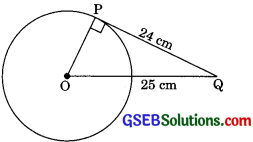QP = 24cm
QO = 25cm .
Also, OP ⊥ PQ
[Radius through the point of contact is perpendicular to the tangent]In right ΔOPQ, by Pythagoras theorem
OQ2 = OP2 + PQ2
252 = OP2 + 24
OP2 = 252 – 242
OP2 = 625 – 576 = 49
OP = 7 cm

Question 2.
In figure, if TP and TQ are the two tangents to a circle with centre O, so that ∠POQ = 110°, then ∠PTQ is equal to
(a) 60°
(b) 70°
(c) 80°
(d) 90°Solution:
(b) ∠OPT = ∠OQT = 90°
through the point of contact]
∠OPT + ∠PTQ + ∠OQT + ∠POQ = 360°
[Sum of all angles of quadrilateral]
⇒ 90° + ∠PTQ + 90° + 110° = 360°
290° + ∠PTQ = 360°
⇒ ∠PTQ = 360° – 290° = 70°

Question 3.
If tangents PA and PB from a point P to a circle with centre O are inclined to each other at an angle of 80°, then ∠POA is equal to:
(a) 50°
(b) 60°
(c) 70°
(d) 80°Solution:
(a) Join OP
In ΔPOA and ΔPOB
PA = PB [Tangents from external point P]
OA = OB
OP = OP
⇒ ΔPOA ≅ ΔPOB [SSS congruency rule]
⇒ ∠OPA = ∠OPB = 40°
Now, in ΔOAP
∠POA + ∠OAP + ∠APO = 180°
[Angle sum property of triangle]
∠POA + 90° + 40° = 180°
∠POA + 130° = 180°
∠POA = 50°Question 4.
Prove that the tangent lines at the ends of a diameter of a circle are parallel.
Solution:
From Fig.∠1 = 90° ……….(1)
[Radius is perpendicular to the tangent at the point of contact]
∠2 = 90° ……….(2)
From (1) and (2),
∠1= ∠2
But these are alternate interior angles.
∴ PQ || RS

Question 5.
Prove that the perpendicular at the point of contact to the tangent to a circle passes through the centre.
Solution:
Given:
A circle with centre O.
AB is a tangent at point A.
O’A ⊥ AB
To prove: AO’ passes through the centre O.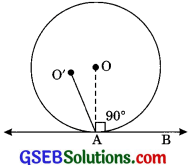Construction: Join OA.
Proof: ∠O’AB = 90° (Given)
∠OAB = 90°
[Radius is perpendicular at the point of contact]
⇒ ∠O’AB = ∠OAB = 90°
which is possible only when O’ and O coincides
with each other.
⇒ AO’ and AO is the same line.
∴ AO’ passes through the centre O.Question 6.
The length of a tangent from a point A at distance 5 cm from the centre of the circle is 4 cm. Find the radius of the circle.
Solution:
∠OPA = 90° [Theorem 10.1]
∴ OA2 = OP2 + AP2 [By Pythagoras theorem]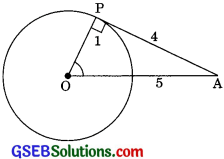52 = OP2 + 42
OP2 = 25 – 16 = 9
OP = $$\sqrt{9}$$ = 3 cm
∴ The radius of the circle is 3 cm.

Question 7.
Two concentric circles are of radii 5 cm and 3 cm. Find the length of the chord of the larger circle which touches the smaller circle.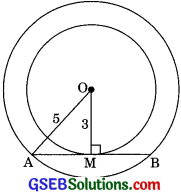Solution:
Let O be the common centre of two concentric circles.
AB, the chord of the larger circle which touches the smaller circle at M.
Join 0M and OA.
Then ∠OMA = 90° [Theorem 10.11]
∴ OMA is a right-angled triangle.
OA2 = OM2 + AM2 [By Pythagoras theorem]
52 + 32 + AM2
25 = 9 + AM2
AM2 = 25 – 9 = 16
AM = $$\sqrt{16}$$ = 4cm
Now, AM = BM = 4 cm
[The perpendicular from the centre of a circle to a chord bisects the chord]
∴ AB = AM + BM
= AM + AM = 2AM
2 x 4 = 8 cmQuestion 8.
A quadrilateral ABCD is drawn to circumscribe a circle (See Figure). Prove that AB + CD = AD +BC.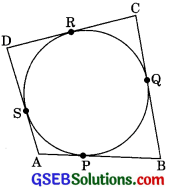[CBSE 2008, 09, 12, 13, 16, 171
Solution:
Since the tangents drawn from an external point are equal in length.
∴ AP = AS ………(1)
BP = BQ ……..(2)
CR = CQ ………(3)
DR = DS ………(4)
Adding (1), (2), (3), (4), we get
(AP + BP) + (CR + DR)
⇒ AS + BQ + CQ + DS
⇒ AB + CD = (AS + DS) + (BQ + CQ)
⇒ AB + CD = AD + BC

Question 9.
In Fig. XY and X’Y’ are two parallel tangents to a circle with centre O and another tangent AB with the point of contact C intersecting XY at A and X’Y’ at B. Prove that ∠AOB = 90°. [CBSE 2011, 2012, 2013, 2017]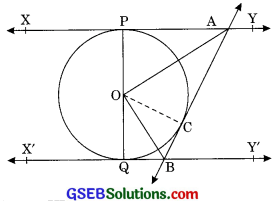Solution:
Given: XY and X’Y’ are two parallel tangents with centre O and AB is another tangent at the point of contact C and intersecting XY at A and X’Y’ at B.
To prove: ∠AOB = 90°
Construction: Join OC.
Proof: ∠OPA = 90° [Theorem 10.11]
∠OCA = 90°
∴ In ΔOPA and ΔOCA
OA = OA (Common)
AP = AC [Tangents drawn from an external point are equal in length ]
and ∠OPA = ∠OCA = 90°
⇒ ΔSOPA ≅ ΔSOCA [RHS congruence criteria]
⇒ ∠OAP = ∠OAC [CPCT]
⇒ ∠OAC = $$\frac {1}{2}$$ ∠PAB
Similarly, ∠OBQ = ∠OBC
⇒ ∠OBC = ∠QBA ……….(2)
∴ XY || XY,
and a transversal AB intersects them
⇒ ∠PAB + ∠QBA = 180° [Sum of the cointerior angles is 180° ]
⇒ $$\frac {1}{2}$$ ∠PAB + $$\frac {1}{2}$$∠QBA = $$\frac {1}{2}$$ x 180°
∠PAB + ∠QBA = 90° ………(3)
[From (1) and (2)]
In ΔAOB
∠OAC + ∠OBC + ∠AOB = 180°
[Angle sum property of triangle]
⇒ 90° + ∠AOB = 180° [From (3)]
∠AOB = 180° – 90° = 90°
∠AOB = 90°Question 10.
Prove that the angle between the two tangents drawn from an external point to a circle is supplementary to the angle subtended by the line segments joining the points of contact at the centre. [CBSE 2012]
Solution:
From Fig.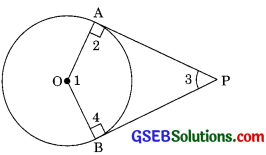∠OPA = 90° ……..(1)
∠OBP = 90 ……….(2)
[Radius is perpendicular to the tangent at the point of contact]
∴ ∠APB + ∠OBP + ∠AOB + ∠OAP = 360°
∠APB + 90° + ∠AOB + 90° = 360°
[From (1) and (2)]
∠APB + ∠AOB = 360° – 180° = 180°
∠APB and ∠AOB are supplementary angles.

Question 11.
Prove that the parallelogram circumscribing a circle is a rhombus. [CBSE 20121
Solution:
Given: ABCD is a parallelogram.
To prove: ABCD is a rhombus.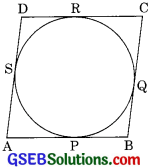Proof: The tangents drawn from an external point are equal in length.
∴ AP = AS …….(1)
PB = BQ ………(2)
CR = CQ ……..(3)
DR = DS ……..(4)
Adding (1), (2), (3), (4), we get
AP + PB + CR + DR = AS + BQ + CQ + DS
⇒ (AP + PB) + (CR + DR) = (AS + DS) + (BQ + CQ)
⇒ AB + CD = AD + BC
⇒ AB + AB = BC + BC
(Opposite sides of parallelogram are equal]
⇒ 2AB = 2BC
⇒ AB = BC
Now AD = BC = AB = CD
⇒ ABCD is a rhombus.Question 12.
A triangle ABC is drawn to circumscribe a circle of radius 4 cm such that the segments BD and DC into which BC is divided by the point of contact D are of lengths 8 cm and 6 cm respectively (See Figure). Find the sides AB and AC.Solution:
Given CD 6 cm
BD = 8 cm and radius = 4 cm
Join OC, OA and OB.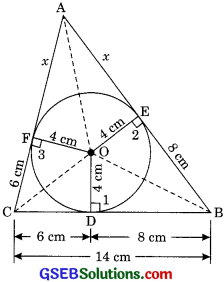As the tangents drawn from an external point are equal in length
∴ CD = CF = 6cm
BD = BE = 8 cm
and let AF = AE = x cm

In ΔOCB,
Area of ΔOCB
= $$\frac {1}{2}$$ base x height
= $$\frac {1}{2}$$ x CB x OD
= $$\frac {1}{2}$$ x 14 x 4 = 28 cm2
Similarly, Area of ΔOCA
= $$\frac {1}{2}$$ x AC x OF
= $$\frac {1}{2}$$ x (6 + x) x 4
= (12 + 2x) cm2
Similarly, Area of ΔOBA
= $$\frac {1}{2}$$ x AB x OE
= (8 + x) x 4
=(16 + 2x) cm2
Now, area of ΔABC
= ar(OCB) + ar(OCA) + ar(OBA)
= 28 + (12 + 2x) + (16 + 2x)
= 56 + 4x …………(1)
Now, perimeter of ΔABC
= AB + BC + CA
= x + 6 + 14 + 8 + x
= 28 + 2x
S = $$\frac {1}{2}$$ (28 + 2x) = 14 + x
By Heron’s formula,
area of ΔABC = $$\sqrt{S(S-a)(S-b)(S-c)}$$
= $$\sqrt{(14 + x)(14 + x – 14)(14 + x – x – 6)(14 + x – x – 8)}$$
= $$\sqrt{(14 + x) \cdot x \cdot(8)(6)}$$
= $$4 \sqrt{3 x(14 + x)}$$ …….(2)
From equation (1) and (2), we get
56 + 4x = $$4 \sqrt{3 x (14 + x)}$$
14 + x = $$\sqrt{3 x(14 + x)}$$
On squaring both sides,
(14 + x)2 = 3x(14 + x)
14 + x = 3x
2x = 14
x = 7
∴ Length AC = 6 + x = 6 + 7 = 13cm
Length AB = 8 + x = 8 + 7 = 15 cmQuestion 13.
Prove that the opposite sides of a quadrilateral circumscribing a circle subtended supplementary angles of the centre of the circle. [CBSE 2012, 2017]
Solution: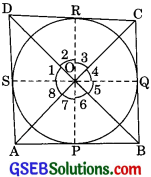Given:
ABCD is a quadrilateral circumscribing a circle whose centre is O.
To prove:
(i) ∠BOC + ∠AOD = 180°
(ii) ∠AOB + ∠COD = 180°
Construction. Join OP, OQ, OR and OS;
where P, Q, R and S are points of contacts of tangents AB, BC, CD and DA to the circle.
Proof:
(i) As tangents drawn from an external point are equal in length.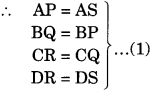In ΔOBP and ΔOBQ
OP = OQ [Radii of the same circle]
OB = OB [Common]
BP = BQ [From(1)]
∴ ΔOBP ≅ ΔOBQ [SSS congruency rule]
∴ ∠5 = ∠6 [CPCT]
Similarly,Now, ∠1 + ∠2 + ∠3 + ∠4 + ∠5 + ∠6 + ∠7 + ∠8 = 360°